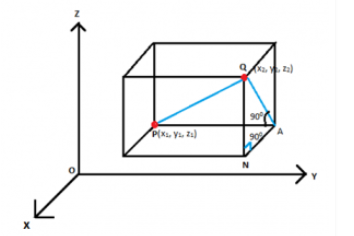# Three Dimensional Geometry

You must have learn about 2-D geometry or two-dimensional coordinate system. Here you will learn about three dimensional geometry and other related topics such as definition, formulas, problems based on it.

## Introduction to Three Dimensional Geometry

When we talk about 2 dimension geometry, it consists of two coordinates say, x and y in the plane. But when we speak about 3 dimensional geometry, it consists of three coordinates say, x, y and z.

Three-dimensional geometry deal with shapes of square, rectangle, cuboid, rhombus, trapezium, parallelogram etc, which has coordinates in three-dimensional space. These shapes or figures have dimensions-length, width and height. You can take examples of 3D geometry from real worlds also.Like, a room of a house has length, width and height as its dimensions.### Three Dimensional Geometry Formulas

#### Distance Formula

Let P and Q are two points in space which has coordinates ($x_{1},y_{1},z_{1}$) and ($x_{2},y_{2},z_{2}$), respectively.The distance between two points P and Q is defined by then,

$PQ=\sqrt{(x_{2}-x_{1})^{2}+(y_{2}-y_{1})^{2}+(z_{2}-z_{1})^{2}}$

#### Section Formula

1. If a point R divides the line segment joining the two points P($x_{1}, y_{1}, z_{1}$) and Q($x_{2}, y_{2}, z_{2}$) in the ratio of m:n, internally, then, coordinates of R are;
$R( \frac{mx_{2}+ nx_{1}}{m+n}, \frac{my_{2}+ ny_{1}}{m+n}, \frac{mz_{2}+ nz_{1}}{m+n})$
1. If a point R divides the line segment joining the two points P($x_{1},y_{1},z_{1}$) and Q($x_{2},y_{2},z_{2}$) in the ratio of m:n, externally, then, coordinates of R are;
$R( \frac{mx_{2}- nx_{1}}{m-n}, \frac{my_{2}- ny_{1}}{m-n}, \frac{mz_{2}- nz_{1}}{m-n})$

#### Mid-Point Formula

If R is the mid-point of the segment joining P($x_{1},y_{1},z_{1}$) and Q($x_{2},y_{2},z_{2}$), then m=n=1 and the coordinate R is given by;

$R( \frac{x_{1}+ x_{2}}{2}, \frac{y_{1}+ y_{2}}{2}, \frac{z_{1}+ z_{2}}{2})$

#### For Centroid of Triangle

Centroid of the triangle whose vertices are ($x_{1},y_{1},z_{1}$), ($x_{2},y_{2},z_{2}$) and ($x_{3},y_{3},z_{3}$), the coordinate R is given by;

$R( \frac{x_{1}+ x_{2}+ x_{3}}{3}, \frac{y_{1}+ y_{2}+ y_{3}}{3}, \frac{z_{1}+ z_{2}+ z_{3}}{3})$

These formulas are the key concepts and are also covered under three dimensional geometry Class 11 syllabus.

#### Three Dimensional Geometry Problem and Solution:

To get a clear understanding of the three dimensional formulas, you should solve three dimensional geometry problems. Let us give an example problem here.

Problem: Find the distance between the points P(-2,4,1) and Q(1,2,-5).

Solution: Here, $x_{1}$=-2, $y_{1}$=4, $z_{1}$=1, $x_{2}$=1, $y_{2}$=2 and $z_{2}$=-5.

By the distance formula, we know,

PQ=$\sqrt{(x_{2}-x_{1})^{2}+(y_{2}-y_{1})^{2}+(z_{2}-z_{1})^{2}}$

PQ=$\sqrt{(x_{2}-x_{1})^{2}+(y_{2}-y_{1})^{2}+(z_{2}-z_{1})^{2}}$

PQ=$\sqrt{(1-(-2))^{2}+(2-4)^{2}+(-5-1)^{2}}$

PQ=$\sqrt{9+4+36}$

PQ=7 units

Similarly, you can also solve more problems on the above-given formulas by defining the values for each quantity.

For geometry related topics, download BYJU’S- The Learning App.

 MATHS Related Links Three Dimensional Shapes Chapter 11 – Three dimensional Geometry Co-ordinate Geometry Coordinate System Class 11 Maths Index